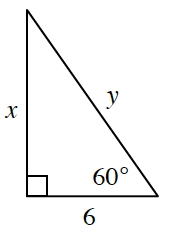### Home > CCG > Chapter 4 > Lesson 4.2.2 > Problem4-74

4-74.Find $x$ and $y$ in the diagram at right. Show all of the steps leading to your answer.

Refer to the Math Notes box in Lesson 4.1.4 to help you solve for $x$.

Now use the Pythagorean Theorem to solve for $y$.

$x\approx10.39$, $y=12$